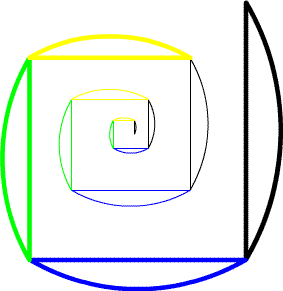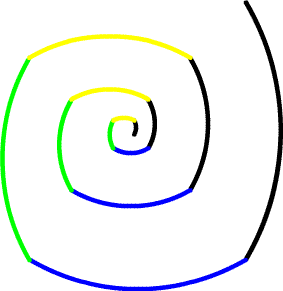Temporary Work _______ 20070418—20070419 This “Hunch” is not really a complete solution, although some things will be discovered. This Hunch’s purpose is to present a challenging math problem. One that I feel has an answer, but the answer is no easy task. This problem is looking for patterns of in prime numbers, a problem which has existed for centuries and has no simple solution. Here is the Problem: Mathematicians have been searching for patterns in prime numbers to see if there is any way to determine where higher prime numbers will occur. This isn’t a difficult task for small numbers but as numbers increase, computers cannot find the answer in short times. So if there was a simple way (although it might not be easy to find), many math problems such as encryption could be solved. So that is the goal–to find an easy way to identify prime numbers. I propose this: We already know many properties of involutes and logarithmic spirals. It would be useful to note what is the absolute length around a logarithmic spiral. (the length of the spiral itself) Well it is difficult to find this value without an equation. But with basic trigonometry we know that [S = r * (angle of rotation in radians)]. So we know certain parts of the logarithmic spiral is based on this rule. (See previous math hunch.) We also know about the integral from calculus. Perhaps there is a way to use the summation of the logarithmic spiral section. —But perhaps we don’t even have to employ such advanced techniques. Since the logarithmic spiral is directly related to an involute, maybe if we create an logarithmic spiral based on the change of prime numbers, we can find a simple way to describe the logarithmic spiral using an involute. (Again see previous math hunch.) Since division is a matrix of values there has to be a pattern in primes due to the fact they are not divisible by any number but themselves and 1. (*This has to be proven.) Logarithmic spirals can show these values. The only problem is describing the logarithmic spiral with an equation. So we must rely on the knowledge of logarithmic spirals, involutes, and parabolas to show special “number theory” relationships.   The grunt work of drawing and solving the logarithmic spiral: A logarithmic spiral needs to be constructed. (See following drawings.) It is broken into several circular segments. For every pi radians the arc length (S) equals the sequence of the prime numbers. For example, we start with an arc length of 2—the following arc is the next prime number 3. To create this drawing a line equal to 2/pi was drawn. Then an arc of radius 0.63662 (which equals r = S/(angle in radians) = 2/pi) was drawn over top the line. This sequence is repeated for each pi radians with the arc with radius— (prime number/pi)— drawn perpendicular to the preceding value. Find the involute that describes this logarithmic spiral and you will have found a way to find prime numbers!!!! references: 1. "Old and New Unsolved Problems in Plane Geometry and Number Theory", Victor Klee--Stan Wagon, (for basic information of primes and help choosing a problem to work on) 2. WikiPedia.org (for list of prime numbers and basic definitions)You can download the file yourself to get exact values. It is an AutoCad 14 dxf. Click Here. Save target as